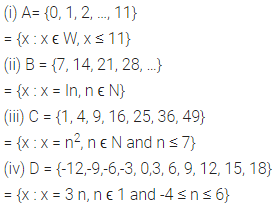# ML Aggarwal Class 6 Solutions for ICSE Maths Chapter 5 Sets Ex 5.1

## ML Aggarwal Class 6 Solutions for ICSE Maths Chapter 5 Sets Ex 5.1

Question 1.
State which of the following collections are set:
(i) collection of odd natural numbers less than 50
(ii) collection of four colours of a rainbow
(iii) collection of the first three days of a week
(iv) collection of all tall students of your class
(v) collection of all clever students of your school
(vi) collection of all rich people of Bangalore
(vii) collection of some multiples of 5 (viii) collection of all prime numbers
(ix) collection of all even integers which lie between -5 and 15
(x) collection of all good cricket players of India
(xi) collection of three youngest students of your class
(xii) collection of three healthy students of your class
Solution: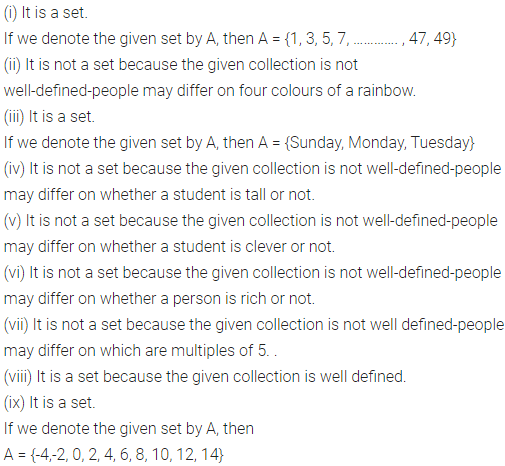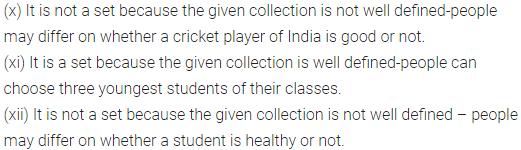Question 2.
Let E = {even integers}. Insert the appropriate symbol ϵ or ∉ in the blanks:
(i) 10 …. E
(ii) -8 …. E
(iii) 13 … E
(iv) {6} …. E
(v) a …. E (vi) -4, 12, …. E
Solution:Question 3.
Let V = {vowels in English alphabet}. Write which of the following statements are true and which are false :
(i) c ϵ V
(ii) {a} ϵ V
(iii) a, e, i ϵ V
(iv) a, b ϵ V
(v) {a, u} ∉ V
(vi) {a, o, u} ϵ V
Solution:Question 4.
Write the following sets in roster form:
(i) the set of first five odd counting numbers
(ii) the set of all even natural numbers less than 101
(iii) {months of year whose names begin with a vowel}
(iv) {one digit natural numbers which are perfect squares}
(v) the set of multiples of 7 which lie between -20 and 25
(vi) {factors of 36}
(vii) {prime factors of 360}
(viii) the set of whole numbers which are multiples of 5
(ix) the set of all letters in the word ‘CHENNAI’
(x) The set of all vowels in the word ‘MUSSOORIE’
(xi) the set of all consonants in the word ‘MATHEMATICS’
Solution:Question 5.
Write the following sets in tabular form:
(i) {x : is a natural number and x < 7}
(ii) {x : x e W and x ≤ 5}
(iii) {x : x is a month.of a year having less than 31 days}
(iv) {x | x is a letter in the word ‘CIRCUMFERENCE’}
(v) {x | x is a vowel in the word ‘NOTATION’}
(vi) (x : x is a digit in the numeral 110526715}
(vii) {x : x is a factor of 48}
(viii) (x : x is a multiple of 11 and 0 ≤ x < 80}
(ix) [y : y is a two digit natural number divisible by 10}
Solution: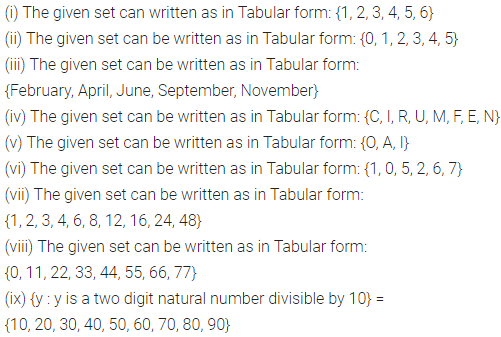Question 6.
Write the following sets in roster form and also in set-builder form:
(i) the set of integers which lie between -2 and 3 (both inclusive)
(ii) the set of letters in the word ‘ULTIMATUM’
(iii) {months of a year whose names begin with J}
(iv) the set of single-digit whole numbers which are perfect squares
Solution:Question 7.
Write the following sets in tabular form and also in descriptive form :
(i) {x : x is a prime number less than 30}
(ii) the set of whole numbers which are multiples of 8 and less than 50
(iii) {x | x is a consonant in the word ‘QUESTION PAPER’}
Solution: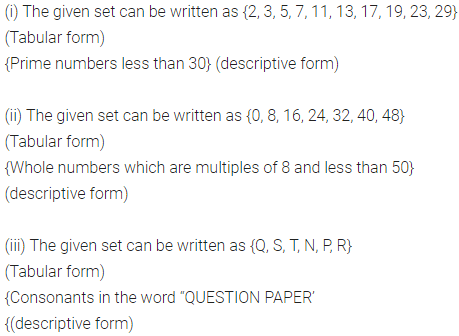Question 8.
Write the following sets in the set builder form:
(i) A= {0, 1, 2, …, 11}
(ii) B = {7, 14, 21, 28, …}
(iii) C = {1, 4, 9, 16, 25, 36, 49}
(iv) D = {-12, -9, -b, -3, 0, 3, 6, 9, 12, 15, 18}
Solution: